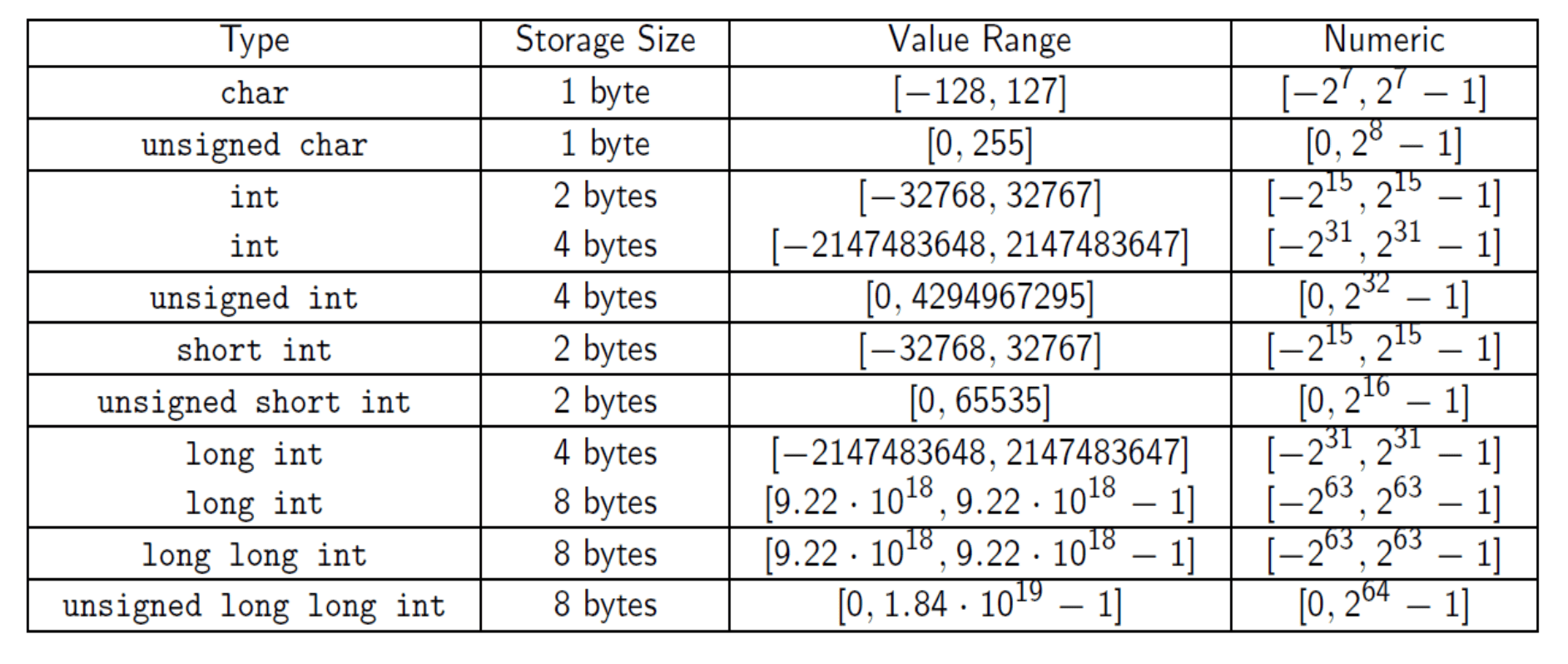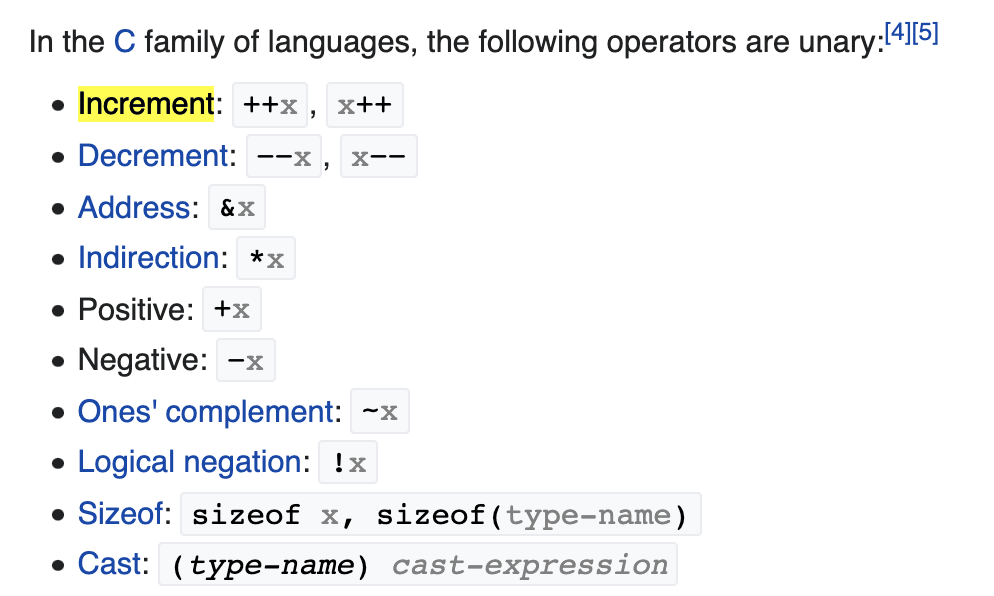# C Language §

The C language is one of the oldest programming languages.

We were taught this at Waterloo because I am in SE, so we can better understand how software communicates with the machine.

Use valgrind ./a.out to check for memory lead.

### Compilation §

gcc prog1.c

By default, it will compile an executable to ./a.out

If you want to specify the output,

gcc prog1.c -o prog1


### Variables §

Rules for names:

• Must begin with a letter or an underscore.
• After the first letter, can be letters, numbers or underscores.
• Case sensitive.
• Cannot be keywords (eg. int, while etc.)### Basic Syntax §

Always end with semi-colons.

#include <stdio.h>

int main(void) {
// Do things here

return 0;
}

### Operators §

Basic Operations

• +/-/* to add/subtract/multiply two values
• / to divide two values (if both values are integers, then the result is integer as well (all decimal portions of the result is lost)
• % to give the remainder of dividing the first value by the second value () can be used as well.

Assignment Operator =

### Unary Operators §#### Increment / Decrement Operators §

• ++a; would increment a by one and then possibly use a.
• --a; would decrement a by one and then possibly use a.
• a++; would use a first (if applicable) then increment a by one.
• a--; would use a first (if applicable) then decrement a by one.

### Modular Programming §

Interface File In C, there exists the interface file (.h) that includes definitions of new data types and function declarations.

Why interface files?

This is often used because we don’t want the client to actually see the logic on the backend, just the interface.

### Array in C §

If you initialize one value, the rest of the array will be 0. Else, all the values of the array will be garbage.

### Math §

Dealing with floats

• Comparing x == y is often risky.
• To be safe, instead of using if (x == y) you can use if (x-y < 0.0001 || y-x < 0.0001) (or use absolute values)
• We sometimes call Ɛ = 0.0001 the tolerance.

Converting decimal to Binary

1. To convert the fractional part to binary, multiply fractional part with 2 and take the one bit which appears before the decimal point.
2. Follow the same procedure with after the decimal point (.) part until it becomes 1.0.

In floating number storage, the computer will allocate 23 bits for the fractional part. So, it’s enough to do the above method at max 23 times.

Math compilation To compile a C program that includes math.h library in Linux you need to add -lm,

gcc  -std=c11 program.c -lm


#### Errors §

Let r be the real number we’re approximating and let p be the approximate value. Absolute error is given by Relative error is given by

### Using C in Python §

Say you wrote some C code and want to run it inside Python. You can actually do that, here are the following steps:

cc -fPIC -shared -o my_functions.so my_functions.c